# Python Program to Check Positive or Negative or Zero

In this article, we've covered some programs in Python, to check whether a number entered by user is a positive or negative number, or zero. Here are the list of programs:

• Simple Program to Check Positive, Zero or Negative Number
• Check Positive, Negative or Zero using Function
• Using Class

## Check Positive, Zero or Negative Number

To check whether a number entered by user at run-time, is a positive, negative, or zero in Python, you have to ask from user to enter a number, and then check and print the message as shown in the program given below:

```print("Enter the Number: ")
num = int(input())

if num<0:
print("\nNegative Number")
elif num==0:
print("\nZero")
else:
print("\nPositive Number")```

Here is its sample run:Now supply the input say 10 as number and press `ENTER` key to check whether it is a positive number, negative number or a zero: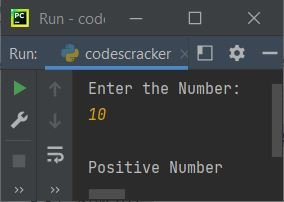Here is another sample run with user input -10: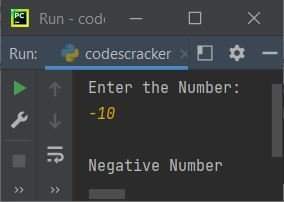#### Modified Version of Previous Program

This is the modified version of previous program. This program outputs data in more generalized way. Here the end is used to skip printing of an automatic newline. And the str() method is used to convert any type of value to a string type.

```print("Enter a Number: ", end="")
n = int(input())

if n<0:
print("\n" +str(n)+ " is a Negative Number")
elif n==0:
print("\n" +str(n)+ " is Zero")
else:
print("\n" +str(n)+ " is a Positive Number")```

Here is its sample run with user input, 0: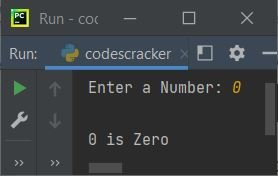## Check Positive, Negative, Zero using Function

This program uses a user-defined function named checkPNZ() that receives a number as its argument. And returns either 1 if it is a positive, or 0 if it is a zero.

Therefore we've passed the number entered by user as its argument, so the return value gets initialized to chk. And through chk's value, we've compared and printed the message as shown here:

```def checkPNZ(x):
if x>0:
return 1
elif x==0:
return 0

print("Enter a Number: ", end="")
n = int(input())

chk = checkPNZ(n)
if chk==1:
print("\n" +str(n)+ " is a Positive Number")
elif chk==0:
print("\nIt is Zero")
else:
print("\n" +str(n)+ " is a Negative Number")```

Here is its sample run with user input, 98: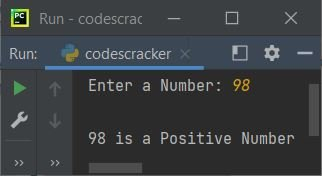The snapshot given below shows another sample run with user input -87: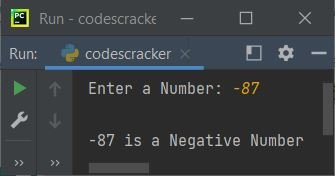## Check Positive, Negative, Zero using Class

This program uses class, an object-oriented feature of Python to do the same job as of previous program.

```class CodesCracker:
def checkPNZ(self, x):
if x>0:
return 1
elif x==0:
return 0

print("Enter a Number: ", end="")
n = int(input())

ob = CodesCracker()
chk = ob.checkPNZ(n)
if chk==1:
print("\n" +str(n)+ " is a Positive Number")
elif chk==0:
print("\nIt is Zero")
else:
print("\n" +str(n)+ " is a Negative Number")```

An object ob is created of class CodesCracker(), to access its member function named checkPNZ() through dot (.) operator. Rest of the things works in similar way as of a normal function.

Python Online Test

« Previous Program Next Program »